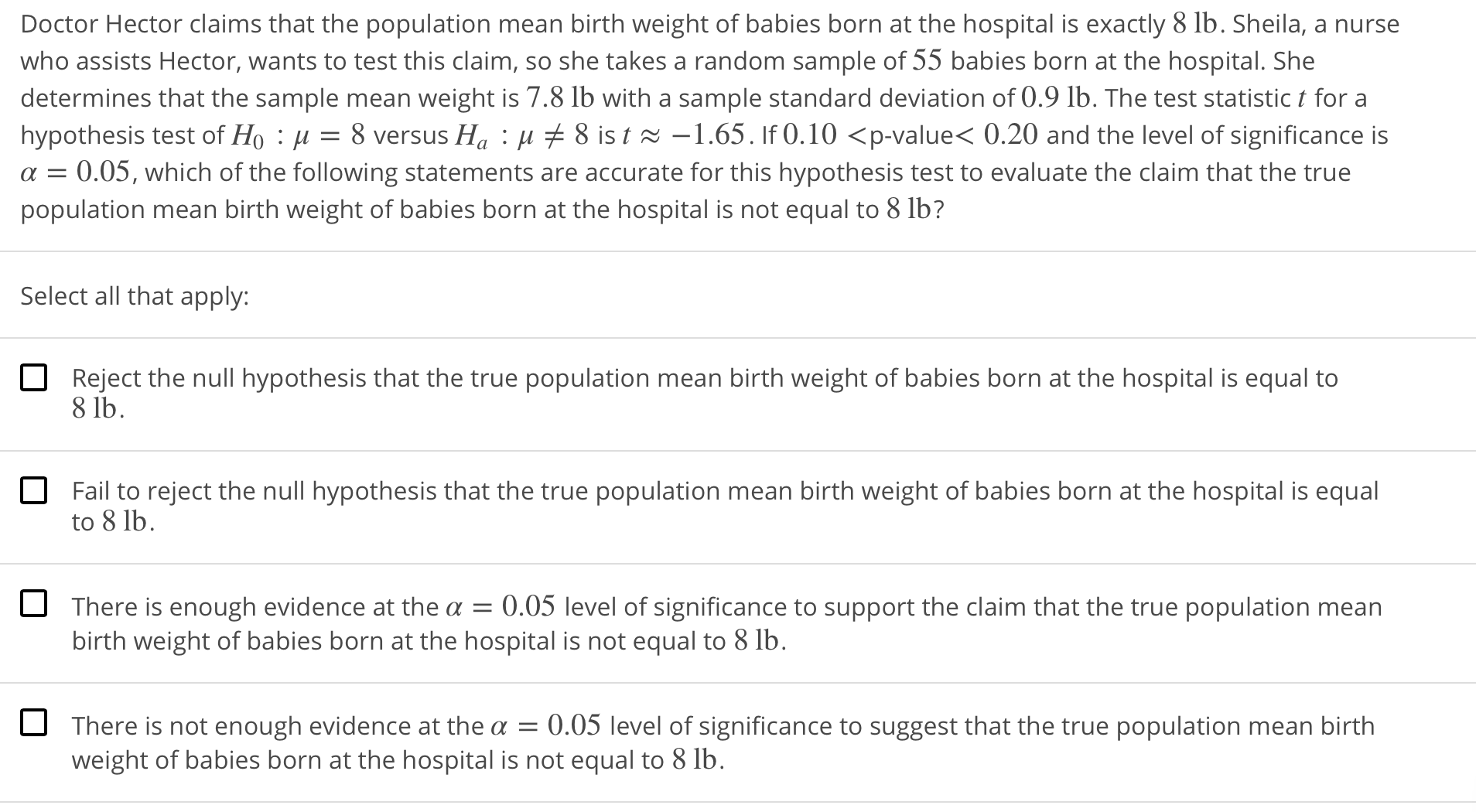Doctor Hector claims that the population mean birth weight of babies born at the hospital is exactly 8 lb. Sheila, a nursewho assists Hector, wants to test this claim, so she takes a random sample of 55 babies born at the hospital. Shedetermines that the sample mean weight is 7.8 lb with a sample standard deviation of 0.9 lb. The test statistic t for ahypothesis test of Ho : μ = 8 versus Ha : μ 8 is t ~一1.65 . If O. 10 〈p-value< 0.20 and the level of significance isα = 0.05, which of the following statements are accurate for this hypothesis test to evaluate the claim that the truepopulation mean birth weight of babies born at the hospital is not equal to 8 lb?Select all that apply:Reject the null hypothesis that the true population mean birth weight of babies born at the hospital is equal to8 lbFail to reject the null hypothesis that the true population mean birth weight of babies born at the hospital is equalto 8 lbOThere is enough evidence at the α-0.05 level of significance to support the claim that the true population meanbirth weight of babies born at the hospital is not equal to 8 lb.There is not enough evidence at the a 0.05 level of significance to suggest that the true population mean birthweight of babies born at the hospital is not equal to 8 lb.

Questionhelp_outlineImage TranscriptioncloseDoctor Hector claims that the population mean birth weight of babies born at the hospital is exactly 8 lb. Sheila, a nurse who assists Hector, wants to test this claim, so she takes a random sample of 55 babies born at the hospital. She determines that the sample mean weight is 7.8 lb with a sample standard deviation of 0.9 lb. The test statistic t for a hypothesis test of Ho : μ = 8 versus Ha : μ 8 is t ~一1.65 . If O. 10 〈p-value< 0.20 and the level of significance is α = 0.05, which of the following statements are accurate for this hypothesis test to evaluate the claim that the true population mean birth weight of babies born at the hospital is not equal to 8 lb? Select all that apply: Reject the null hypothesis that the true population mean birth weight of babies born at the hospital is equal to 8 lb Fail to reject the null hypothesis that the true population mean birth weight of babies born at the hospital is equal to 8 lb O There is enough evidence at the α-0.05 level of significance to support the claim that the true population mean birth weight of babies born at the hospital is not equal to 8 lb. There is not enough evidence at the a 0.05 level of significance to suggest that the true population mean birth weight of babies born at the hospital is not equal to 8 lb. fullscreen
Step 1

A hypothesis test is carried away to check the claim that mean weight of babies born at hospital is exactly 8 lbs.

In a hypothesis test if the p value is less than the level of significance then we have sufficient evidence at given level ...

Want to see the full answer?

See Solution

Want to see this answer and more?

Our solutions are written by experts, many with advanced degrees, and available 24/7

See Solution
Tagged in

Hypothesis Testing